# Clutters III

A long time ago I started a series of posts abut clutters. The most recent post followed the textbook by Gérards Cornuéjols in defining several important classes, and introduced a Venn diagram, showing the relationships between them.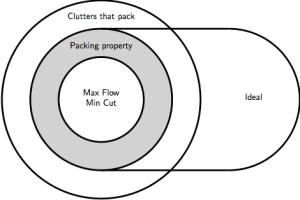In this post we will spend a little more time discussing this diagram.

We let $H=(S,\mathcal{A})$ be a clutter. This means that $S$ is a finite set, and the members of $\mathcal{A}$ are subsets of $S$, none of which is properly contained in another. We let $M$ stand for the incidence matrix of $H$. This means that the columns of $M$ are labelled by the elements of $S$, and the rows are labelled by members of $\mathcal{A}$, where an entry of $M$ is one if and only if the corresponding element of $S$ is contained in the corresponding member of $\mathcal{A}$. Any entry of $M$ that is not one is zero. Let $w$ be a vector in $\mathbb{R}^{S}$ with non-negative values. We have two fundamental linear programs:

(1) Find $x\in \mathbb{R}^{S}$ that minimises $w^{T}x$ subject to the constraints $x\geq \mathbf{0}$ and $Mx\geq \mathbf{1}$.

The vectors $\mathbf{0}$ and $\mathbf{1}$ have all entries equal to zero and one, respectively. When we write that real vectors $a$ and $b$ with the same number of entries satisfy $a\geq b$, we mean that each entry of $a$ is at least equal to the corresponding entry in $b$.

(2) Find $y\in\mathbb{R}^{\mathcal{A}}$ that maximises $y^{T}\mathbf{1}$ subject to the constraints $y\geq \mathbf{0}$ and $y^{T}M\leq w$.

Lemma 1. Any clutter with the Max Flow Min Cut property also has the packing property.

Proof. Let $H$ be a clutter with the Max Flow Min Cut property. This means that for any choice of vector $w$ with non-negative integer entries, the programs (1) and (2) both have optimal solutions with integer values.

We will show that $H$ has the packing property. According to the definition in the literature, this means that for any choice of vector $w$ with entries equal to $0$, $1$, or $+\infty$, there are optimal solutions to (1) and (2) with integer entries. I think there is a problem with this definition. Assume that $r$ is a row of $M$, and every member of the support of $r$ receives a weight of $+\infty$ in the vector $w$. Then (2) cannot have an optimal solution. If $y$ is a purported optimal solution, then we can improve it by adding $1$ to the entry of $y$ that corresponds to row $r$. We are instructed that if entry $i$ in $w$ is $+\infty$, then this means when $x$ is a solution to (1), then entry $i$ of $x$ must be $0$. Again, we have a problem, for if $r$ is a row of $M$, and the entire support of $r$ is weighted $+\infty$, then (1) has no solution: if $x$ were a solution, then it would be zero in every entry in the support of $r$, meaning that $Mx$ has a zero entry.

The literature is unanimous in saying that $H$ has the packing property if (1) and (2) both have integral optimal solutions for any choice of vector $w$ with entries $0$, $1$, and $+\infty$. As far as I can see, this means that any clutter with $\mathcal{A}$ non-empty does not have the packing property: simple declare $w$ to be the vector with all entries equal to $+\infty$. Then neither (1) nor (2) has an optimal solution at all. I think the way to recover the definition is to say that whenever $w$ with entries $0$, $1$, or $+\infty$ is chosen in such a way that (1) and (2) have solutions, they both have optimal solutions that are integral. This is the definition that I will use here.

After this detour, we return to our task, and assume that $H$ has the Max Flow Min Cut property. Assume that $w$ is a vector with entries equal to $0$, $1$, or $+\infty$, and that (1) and (2) both have solutions. This means that any row in $M$ has a member of its support which is not weighted $+\infty$ by $w$. We obtain the vector $u$ by replacing each $+\infty$ entry in $w$ with an integer that is greater than $|S|$. Now because $H$ has the Max Flow Min Cut property, it follows that there are optimal integral solutions, $x$ and $y$, to (1) and (2) (relative to the vector $u$). We will show that $x$ and $y$ are also optimal solutions to (1) and (2) relative to the vector $w$.

We partition $S$ into $S_{0}$, $S_{1}$, and $S_{+\infty}$ according to whether an element of $S$ receives a weight of $0$, $1$, or $+\infty$ in $w$. We have assumed that no member of $\mathcal{A}$ is contained in $S_{+\infty}$. We note that if $z\in \mathbb{Z}^{S}$ is a vector which is equal to zero for each element of $S_{+\infty}$, and one everywhere else, then $z$ is a solution to (1), by this assumption. Moreover, $w^{T}z=u^{T}z\leq |S|$. Now it follows that $x$ must be zero in every entry in $S_{+\infty}$, for otherwise $u^{T}x>|S|$, and therefore $x$ is not an optimal solution to (1) relative to $u$. Since $x$ is integral and optimal, it follows that we can assume every entry is either one or zero. If $x$ is not an optimal solution to (1) relative to $w$, then we let $z$ be an optimal solution with $w^{T}z < w^{T}x$. But by convention, $z$ must be zero in every entry of $S_{+\infty}$. Therefore $u^{T}z=w^{T}z < w^{T}x=u^{T}z$, and we have a contradiction to the optimality of $x$. Thus $x$ is an optimal solution to (1) relative to the $\{0,1,+\infty\}$-vector $w$.

Now for problem (2). Since $y$ is integral and non-negative, and $y^{T}M\leq w$, where every member of $\mathcal{A}$ contains an element of $S_{0}$ or $S_{1}$, it follows that each entry of $y$ must be either one or zero. Let $z$ be any solution of (2) relative to $w$. Exactly the same argument shows that each entry of $z$ is between zero and one. Therefore $z^{T}\mathbf{1}\leq y^{T}\mathbf{1}$ so $y$ is an optimal solution to (2).

We have shown that relative to the vector $w$, both (1) and (2) have optimal solutions that are integral. Hence $H$ has the packing property. $\square$

Lemma 2. A clutter with the packing property packs.

Proof. This one is easy. In order to prove that $H$ packs, we merely need to show that (1) and (2) have optimal integral solutions when $w$ is the vector with all entries equal to one. But this follows immediately from our revised definition of clutters with the packing property. $\square$

The final containment we should show is that clutters with the packing property are ideal. Idealness means that (1) has an optimal integral solution for all vectors $w\in \mathbb{R}^{S}$. This proof is difficult, so I will leave it for a future post. Usually we prove it by using a theorem due to Lehman [Leh].

Theorem (Lehman). The clutter $H$ is ideal if and only if (1) has an optimal integral solution for all choices of vector $w\in\{0,1,+\infty\}^{S}$.

Question. Is there a short proof that clutters with the packing property are ideal? One that does not rely on Lehman’s (quite difficult) theorem?

We will conclude with some examples showing that various containments are proper.

Let $C_{3}^{2}$ and $C_{3}^{2+}$ be the clutters with incidence matrices
$\begin{bmatrix} 1&1&0\\ 0&1&1\\ 1&0&1 \end{bmatrix} \quad\text{and}\quad \begin{bmatrix} 1&1&0&1\\ 0&1&1&1\\ 1&0&1&1 \end{bmatrix}.$
Let $Q_{6}$ and $Q_{6}^{+}$ be the clutters with incidence matrices
$\begin{bmatrix} 1&1&0&1&0&0\\ 1&0&1&0&1&0\\ 0&1&1&0&0&1\\ 0&0&0&1&1&1 \end{bmatrix} \quad\text{and}\quad \begin{bmatrix} 1&1&0&1&0&0&1\\ 1&0&1&0&1&0&1\\ 0&1&1&0&0&1&1\\ 0&0&0&1&1&1&1 \end{bmatrix}$

Exercise. Check that:

1. $C_{3}^{2}$ is not ideal and does not pack,
2. $C_{3}^{2+}$ packs, but is not ideal,
3. $Q_{6}$ is ideal, but does not pack,
4. $Q_{6}^{+}$ is ideal and packs, but does not have the packing property.

This leaves one cell in the Venn diagram without a clutter: the clutters with the packing property that do not have the Max Flow Min Cut property. In fact, Conforti and Cornuéjols [CC] have speculated that no such clutter exists.

Conjecture (Conforti and Cornuéjols). A clutter has the packing property if and only if it has the Max Flow Min Cut property.

[CC] M. Conforti and G. Cornuéjols, Clutters that Pack and the Max Flow Min Cut Property: A Conjecture, The Fourth Bellairs Workshop on Combinatorial Optimization, W.R. Pulleyblank and F.B. Shepherd eds. (1993).

[Leh] A. Lehman, On the width-length inequality. Mathematical Programming December 1979, Volume 16, Issue 1, pp 245–259.

# SiGMa 2017 Recap

The Workshop on Structure in Graphs and Matroids (SiGMa 2017) just wrapped up a few weeks ago in Waterloo.  This was a continuation of similar workshops organized by Bert Gerards in 20082010, and 2012 and by Stefan van Zwam and Rudi Pendavingh in 2014 and 2016.

I would like to thank Jim Geelen, Peter Nelson, Luke Postle, and Stefan van Zwam (the organizers of this year’s workshop) who did an excellent job in choosing the program and making sure everything ran smoothly.  They even helped fellow Matroid Union blogger Nathan Bowler overcome Canadian Visa issues in time to give his (very nice) plenary talk (thanks also go to Alan).

This year the workshop was held in commemoration of William T. Tutte, who would have turned 100 this year.  See here for a short biography of Professor Tutte.  Some matroid theorists may not be aware of Tutte’s extremely important contributions as a code breaker at Bletchley Park during the Second World War.  The C&O Department at Waterloo has also been hosting a Distinguished Lecture Series in honour of Tutte this summer.  Click on this link for the list of speakers and to watch the videos on YouTube. SiGMa 2017 also featured a rare conference dinner speech by Jim Geelen on Tutte’s work.

In case you were not able to attend, all the talks from SiGMa 2017 were recorded and are now viewable on the C&O Department’s YouTube Channel.  The overall quality of talks was very high.  I won’t bias you with all of my personal favourites, but for example, all the plenaries and Paul Seymour were excellent.

# The Template Theorem

Geelen, Gerards, and Whittle are still working on the write-up of their Herculean effort to build a matroid minor structure theory generalizing the work that Robertson and Seymour did for graphs. One of the consequences of their work has been published, namely a discussion of the highly connected members of a minor-closed class of matroids . This work was mentioned by Peter in passing here and in more detail here. What Peter didn’t talk about was the most detailed version of that structure theorem. Today I will do just that. To keep things light, I will focus on binary matroids, but  has results for matroids representable over any finite field.

## 1. Low-rank perturbations

The key concept is that of a perturbation of a representable matroid. If $M = M[A]$, and $T$ is a matrix with the same dimensions as $A$, then $M[A+T]$ is a perturbation of $M$. The hope is that, if we know a lot about $M$ and $T$ has low rank, then the resulting matroid still resembles $M$ to a large extent. We will study perturbations of graphic matroids, and start with a few examples. It will be convenient to drop the restriction that the perturbed matroid has the same size as $M$, and therefore we will allow the addition of a bounded number of elements.

### 1.1 Adding a row

Let $A$ be the vertex-edge incidence matrix of a graph, and let $A’$ be the matrix obtained from $A$ by adding an arbitrary row. The matroid $M[A’]$ is known as an even-cycle matroid. It is an instance of the class of lift matroids frequently discussed on this blog, such as by Irene (here,  here, and here) and two weeks ago by Daryl (here). Note that it can be obtained from $M$ by coextending the matroid by one element, and then deleting that element. They can be visualized by coloring the edges of the graph, calling an edge even if its corresponding column in the matrix has a 0 in the new row, and odd if it has a 1. This class of matroids is closed under minors.

### 1.2 Adding a column

Let $A$ again be the vertex-edge incidence matrix of a graph, and this time let $A’$ be the matrix obtained from $A$ by adding an arbitrary column. The matroid $M[A’]$ will have one extra element, and is known as a graft. Grafts played a crucial role in the proofs of many fundamental results in matroid theory, including Seymour’s Decomposition Theorem for regular matroids . They can be visualized by coloring the vertices of the graph whose corresponding matrix row has a 1 in the new column.

Note that the class of grafts is not closed under minors (contracting the graft element destroys the graphic structure). However, a closely related class, where we add an element to the graphic matroid and immediately contract that element, is closed under minors. The duals of these matroids are known as the even cut matroids. They have been extensively studied by Guenin, Pivotto, and Wollan (see, for instance, Irene’s PhD thesis ).

### 1.3 Adding a few elements to a low-rank flat

Let $G$ be a graph with $t$ distinguished vertices. We take the vertex-edge incidence matrix, and add a few columns, but now these columns are only allowed to have nonzero entries corresponding to the $t$ distinguished vertices. The resulting class of matroids will resemble a graphic matroid everywhere but in a low-rank set. Note that this can be seen as a variation of the “adding a column” construction, but sometimes it is useful to consider the operation separately.

## 2. Frame templates

Next, we ask ourselves what happens if we allow several of the above operations in a row. Can there be fundamentally different ways to perturb a graphic matroid? Geelen, Gerards, and Whittle answered this question in full generality through the introduction of templates.

Definition. A (binary) frame template is a tuple $\Phi = (C, X, Y_0, Y_1, A_1, \Delta, \Lambda)$ with the following elements:

1. Finite pairwise disjoint sets $C, X, Y_0, Y_1$;
2. A matrix $A_1$ with rows labeled by $X$ and columns labeled by $Y_0\cup Y_1 \cup C$;
3. A set of row vectors $\Delta$ closed under addition, with entries labeled by $Y_0 \cup Y_1 \cup C$;
4. A set of column vectors $\Lambda$ closed under addition, with entries labeled by $X$.

Definition. A matrix $A’$ is said to respect the template $\Phi$ if it is of this form: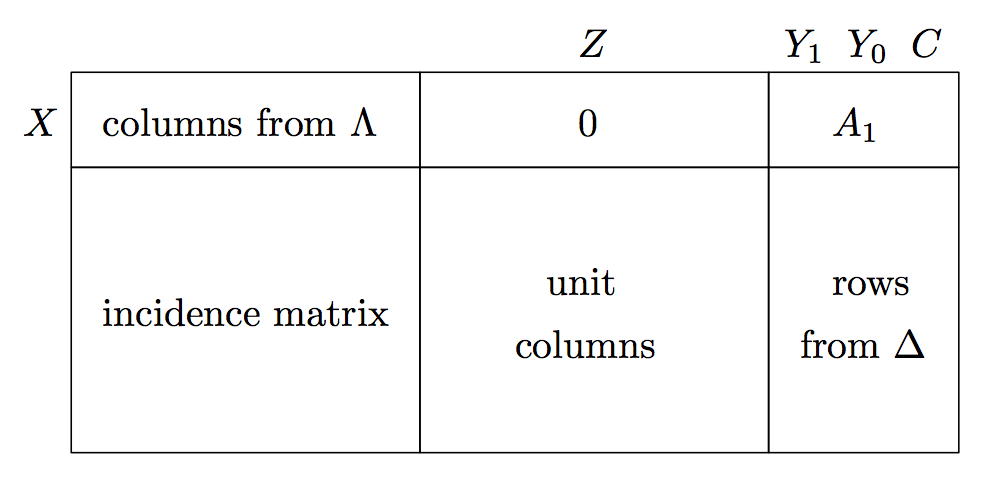Here, a unit column is a column with exactly one nonzero. The incidence matrix and the columns labeled by $Z$ can be of arbitrary size.

Definition. Let $A’$ be a matrix respecting the template $\Phi$. Let $A$ be obtained from $A’$ by

1. Taking each column from $Z$ and adding to it some column labeled by an element of $Y_1$;
2. Deleting the columns labeled by $Y_1$;
3. Contracting the elements labeled by $C$ in the corresponding matroid.

Then $A$ and $M[A]$ are said to conform to the template $\Phi$.

One should think about templates as recipes for constructing families of matroids.

Exercise. Describe the examples from sections 1.1 – 1.3 using templates.

The main result from  is that templates can be used to describe all sufficiently large, sufficiently highly connected matroids in any minor-closed class. Highly connected means the following:

Definition. A matroid $M$ is vertically $k$-connected if, for all separations $(X,Y)$ with $\lambda(X) < k$, either $r(X) = r(M)$ or $r(Y) = r(M)$. In other words, one side of the separation is spanning.

The precise result is:

Theorem (Geelen, Gerards, Whittle ). Let $\mathcal{M}$ be a proper minor-closed class of binary matroids. There exist constants $k, l$ and frame templates $\Phi_1, \ldots, \Phi_t, \Psi_1, \ldots, \Psi_s$ such that:

1. Every matroid conforming to $\Phi_i$ is in $\mathcal{M}$ for $i = 1, \ldots, t$;
2. Every matroid whose dual conforms to $\Psi_j$ is in $\mathcal{M}$ for $j = 1, \ldots, s$;
3. For every vertically $k$ connected matroid $M \in \mathcal{M}$ with at least $l$ elements, there either exists an $i$ such that $M$ conforms to $\Phi_i$ or a $j$ such that $M^*$ conforms to $\Psi_j$.

The third property says that the structure of the highly connected matroids in the class is completely described by a finite list of templates. The first two properties put some quite strict constraints on those templates: any matroid we can build using the template must be a member of the class!

The third property is reminiscent of the result by Robertson and Seymour that the “torsos” of a tree-decomposition are nearly-embeddable in some surface of bounded genus (see [4, Theorem 12.6.6]). But in the graph minors theorem there is no analog of the converse: most graphs nearly-embeddable on that surface won’t be members of the class being studied.

The first two properties can be used to determine the full set of templates $\Phi_1, \ldots, \Phi_t, \Psi_1, \ldots, \Psi_s$ for a given class of matroids. I have worked on several such results with my PhD student Kevin Grace [5, 6]. For instance, let’s consider Seymour’s 1-flowing conjecture, discussed by Dillon here. With Kevin I proved the following:

Theorem (Grace, vZ ). There exist constants $k, l$ such that every vertically $k$-connected 1-flowing matroid on at least $l$ elements is either graphic or cographic.

In other words, any counterexample to Seymour’s conjecture will have to be small, or have a low-order vertical separation. The proof proceeds by considering an arbitrary frame template, and showing that it either has to be trivial, or it can be used to build an excluded minor for the class of 1-flowing matroids. In the process we develop some tools to help with proofs by induction on templates, and to clean up the matrix $A_1$, but those will have to wait for another day.

Other applications of templates include growth rate results, and finding sufficient sets of excluded minors to characterize the highly connected members of a minor-closed class of matroids.

#### References

1. J. Geelen, B. Gerards and G. Whittle, The highly connected matroids in minor-closed classes, Ann. Comb. 19 (2015), 107–123.
2. P. D. Seymour, Decomposition of regular matroids, J. Combin. Theory Ser. B 28(3) (1980), 305-359.
3. I. Pivotto, Even Cycle and Even Cut Matroids. PhD Thesis, University of Waterloo (2011).
4. R. Diestel, Graph Theory, Springer GTM 173, 5th edition (2016).
5. K. Grace, S. H. M. van Zwam, Templates for Binary Matroids, SIAM J. Disc. Mathem. 31(1) (2017), 254 — 282.
6. K. Grace, S. H. M. van Zwam, The highly connected even-cycle and even-cut matroids, Submitted (2016).

# Three classes of graphical matroids

Guest post by Daryl Funk.

Biased graphs, and the frame and lifted-graphic (or simply, lift) matroids associated with them, have been discussed several times already in The Matroid Union blog. Irene Pivotto introduced them in a series of posts, Biased graphs and their matroids I, II, and III. In part II, Irene offered to put money on the truth of the following two conjectures.

Conjecture 1. The class of frame matroids has only a finite number of excluded minors.

Conjecture 2. The class of lift matroids has only a finite number of excluded minors.

If anyone took her up on her offer, you may now collect on your bet. In [CG17], Rong Chen and Jim Geelen exhibit an infinite family of excluded minors for the class of frame matroids, and another for the class of lift matroids. (It is unfair of me to pick on Irene like this — last I heard, bookies were giving 10:1 odds on). These families of excluded minors belong to a third class of matroids having graphical-type structure. So before discussing Rong and Jim’s counter-examples to Conjectures 1 and 2, we had better learn about this new class.

# Quasi-graphic matroids

In his post, Graphical representations of matroids, Jim Geelen discussed a preliminary formulation for a new class of “graphical” matroids, which he there called framework matroids. The goal was to define a single minor-closed class that contains both the classes of frame and lift matroids, and to do so in a way such that (1) the new class maintains or captures the fundamental underlying graphic structure of these matroids, and (2) recognising membership in the new class is tractable — that is, there should be a polynomial-time algorithm to test membership via a rank oracle.

Jim’s goal has largely been realised, with his introduction, along with Bert Gerards and Geoff Whittle, of the class of quasi-graphic matroids, in [GGW17]. There should certainly be a post wholly devoted to this wonderful class of matroids soon. Here, I will tantalise you with just the definition and an example.

Let $M$ be a matroid. A graph $G$ is a framework for $M$ if

1. $E(G)=E(M)$,
2. for each component $H$ of $G$, $r_M(E(H)) \leq |V(H)|$,
3. for each vertex $v$ of $G$, $\operatorname{cl}_M(E(G-v)) \subseteq E(G-v) \cup \{e: e\ \text{is a loop incident to}\ v\},$ and
4. for each circuit $C$ of $M$, the subgraph $G[C]$ induced by $C$ has at most two components.

A matroid is quasi-graphic if it has a framework. The definition is motivated by the following theorem of Paul Seymour, which yields a polynomial-time algorithm to test, via a rank oracle, if a given matroid is graphic. A star of a graph $G$ is the set of edges incident to a vertex $v \in V(G)$ each of whose other endpoint is in $V(G)-v$ (so while $G$ may have loops, loops are not included in any star); $c(G)$ denotes the number of components of $G$.

Theorem 1 [S81]. Let $M$ be a matroid, and let $G$ be a graph. Then $M$ is the cycle matroid of $G$ if and only if

1. $E(G)=E(M)$,
2. $r(M) \leq |V(G)| – c(G)$, and
3. every star of $G$ is a union of cocircuits of $M$.

One can readily see that the requirements for a framework are inspired by the conditions of Theorem 1. One can also see that generalising these conditions to encompass a larger minor-closed class that includes all frame and lift matroids, is not quite straightforward (hopefully, we may learn more about this in a future post). In [GGW17], Jim, Bert, and Geoff show (among other things) that the class of quasi-graphic matroids has the following nice properties:

• It is minor-closed.
• If $(G,\mathcal B)$ is a biased graph, then $G$ is a framework for the lift matroid $LM(G,\mathcal B)$, and $G$ is a framework for the frame matroid $FM(G,\mathcal B)$; thus all lift and all frame matroids are quasi-graphic.
• Given a 3-connected matroid $M$ and a graph $G$, one can check in polynomial time whether $G$ is a framework for $M$.

Jim, Bert, and Geoff conjecture in [GGW17] that there is a polynomial-time algorithm to test, via a rank oracle, if a given matroid is quasi-graphic. In contrast, Rong Chen and Geoff Whittle have recently shown that for each of the classes of frame and lift matroids, testing for membership in the class is intractable [CW16]. More on this in a moment. But first, let us try to get a bit of a feel for what a typical quasi-graphic matroid might look like.

Let us recall some required preliminary concepts. Every frame and every lift matroid may be represented by a biased graph $(G,\mathcal B)$ with $E(G)=E(M)$. For clarity’s sake, I’ll reserve the word circuit for matroids, and use the word cycle for a 2-regular connected subgraph. Recall how the circuits of frame and lift matroids appear in their biased graph representations: they are precisely the edge sets of subdivisions of certain biased subgraphs. Recall, a cycle $C$ of a graph whose edge set is a circuit of the matroid is balanced; otherwise $E(C)$ is independent and $C$ is said to be unbalanced. The figures in Sections 2 and 3 of Irene’s first post on biased graphs, reproduced here for your convenience, illustrate these biased subgraphs.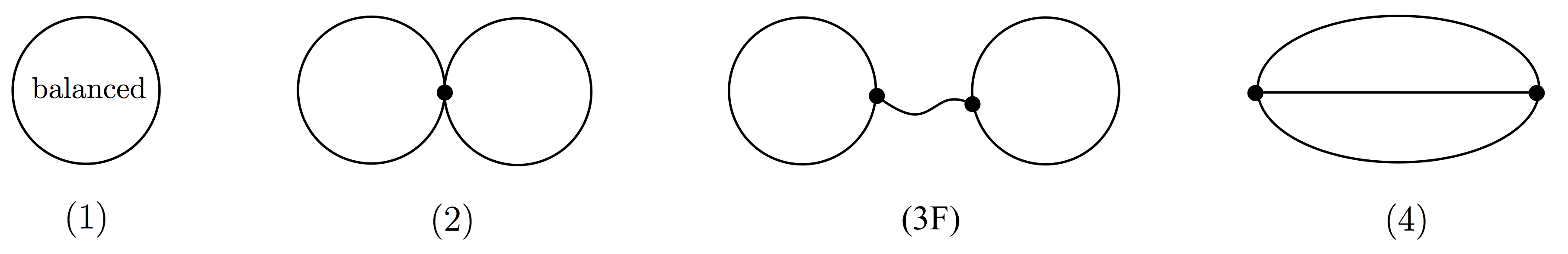Circuits of frame matroids are subdivisions of these graphs. Unlabelled cycles are unbalanced.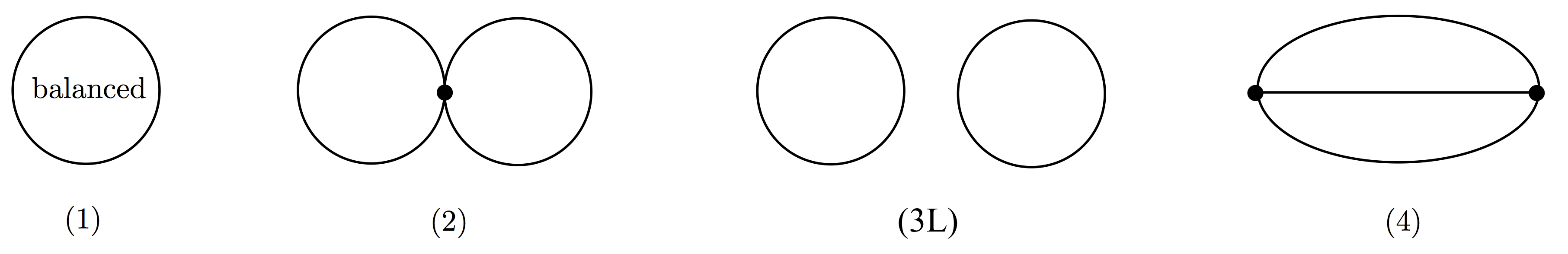Circuits of lift matroids are subdivisions of these graphs. Unlabelled cycles are unbalanced.

Let us call a subdivision of one of these five biased subgraphs (1), (2), (3F), (3L), (4), a circuit-subgraph. Note that the frame and lift matroids associated with a given biased graph differ on just one pair of these circuit-subgraphs, namely, (3F) and (3L) — a pair of vertex disjoint unbalanced cycles forms a circuit of the lift matroid, but is independent in the frame matroid. As Irene has explained in her previous posts, given a biased graph, we get a frame matroid by taking as circuits just circuit-subgraphs of the forms (1), (2), (3F), and (4), we get a lift matroid by taking as circuits just circuit-subgraphs of the forms (1), (2), (3L), and (4), and Tom Zaslavsky has shown that in fact all frame matroids, and all lift matroids, are obtained this way.

What about quasi-graphic matroids? Here is an example. The Vámos matroid (shown below left as a cube, in which the only 4-circuits are the 4 “sides” and just the one “diagonal” plane 2468) is neither a frame matroid, nor a lift matroid, but it is quasi-graphic, with the graph below right providing a framework. (Check that it satisfies the definition!)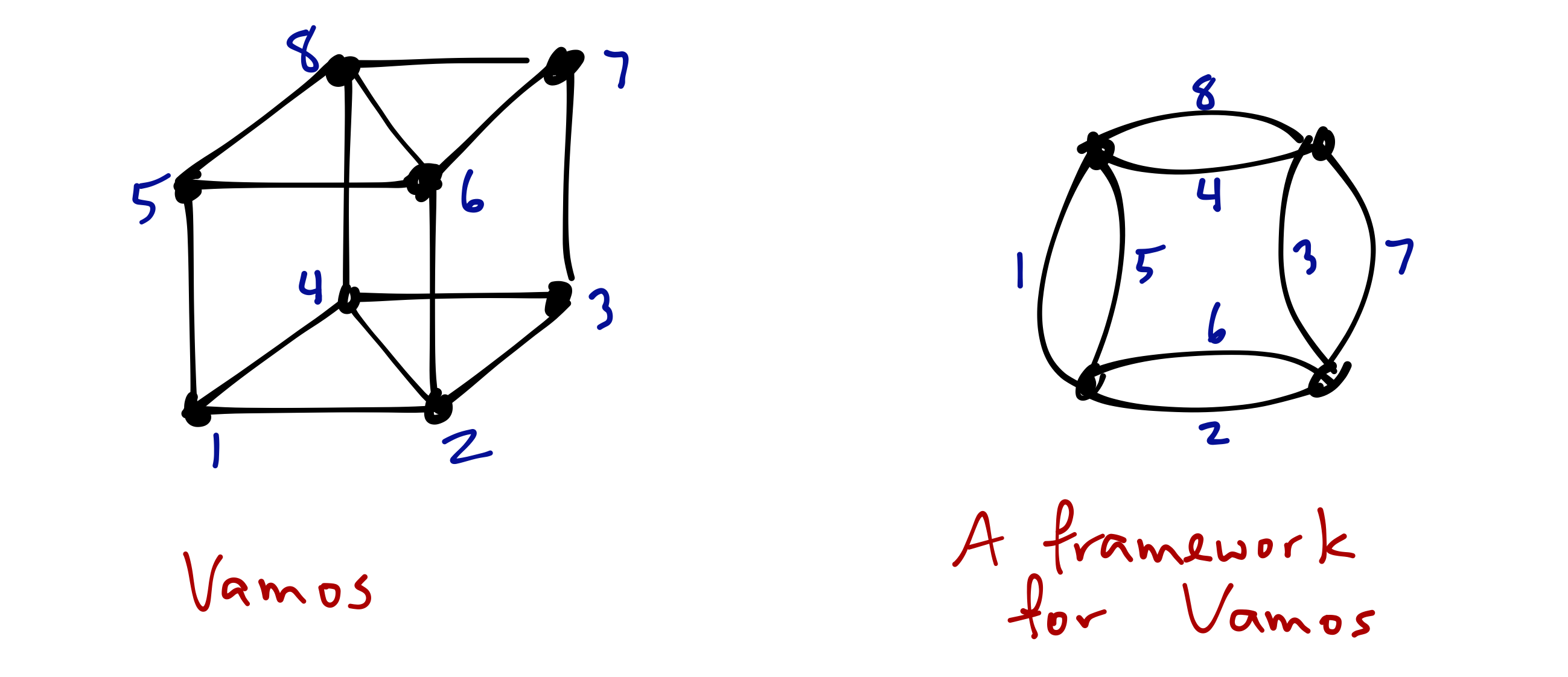Consider the 4-circuits of Vámos, and the subgraphs they form in the framework graph.
The four planes given by the front, back, and sides of the cube each form a circuit-subgraph of type (2), which is a circuit-subgraph for both frame and lift matroids. But together the circuit 2468 and the independent set 1357 prevent this graph from being either a frame or a lift representation for Vámos: circuit 2468 appears as a type (3L) circuit-subgraph, but so does the independent set 1357.

It turns out that (here I’m summarising results of [GGW17]), just as with biased graph representations of frame and lift matroids, the edge set of every cycle in a framework $G$ for a quasi-graphic matroid $M$ is either a circuit of $M$ or is independent in $M$. Further, declaring each cycle of $G$ to be balanced or unbalanced accordingly, just as for frame and lift matroids, yields a biased graph $(G,\mathcal B)$, where $\mathcal B$ denotes the collection of balanced cycles of $G$ (that is, the collection of balanced cycles satisfies the theta property: no theta subgraph contains exactly two balanced cycles). Moreover, every circuit of $M$ appears in $G$ as one of our five circuit-subgraphs (1), (2), (3F), (3L), (4). Conversely, the edge set of each circuit-subgraph of $G$ of one of the forms (1), (2), or (4) is a circuit of $M$, and each of the form of (3F) is either a circuit or contains a circuit of the form (3L).

Thus frameworks for matroids behave very much like biased graph representations for frame and lift matroids. Given a biased graph, taking $\{(1),(2),(3$F$),(4)\}$ as our circuit-subgraphs gives us a frame matroid, taking $\{(1),(2),(3$L$),(4)\}$ as our circuit-subgraphs gives us a lift matroid; and allowing all of $\{(1), (2), (3$F$), (3$L$), (4)\}$ as circuit-subgraphs gives the class of quasi-graphic matroids. This phenomenon is illustrated in the framework for the Vámos matroid above: 2468 is a (3L) circuit-subgraph, while each of the four circuits $1357 \cup e$ for $e \in \{2,4,6,8\}$ are (3F) circuit-subgraphs. Put another way, if a matroid $M$ has a framework having no circuit-subgraphs of type (3F), then we have a biased graph representation for $M$ as a lift matroid; if $M$ has a framework with no circuit-subgraphs of type (3L), then we have a biased graph representation for $M$ as a frame matroid; the Vámos matroid shows that (3F) and (3L) type circuit-subgraphs can coexist in a framework.

# Recognition of frame, and of lift matroids, is intractable

As mentioned above, Rong and Geoff have shown that there can be no algorithm that can determine, via a rank oracle, in time polynomial in the size of the ground set, whether or not a given matroid is a frame matroid. They also show no such algorithm can exist for recognising lift matroids. They do so using two particular families of quasi-graphic matroids, one for frame and one for lift, arising from the same infinite family of framework graphs. More precisely, they prove the following two theorems.

Theorem 2 [CW16]. For any polynomial $p(\cdot)$, there is a frame matroid $M$ such that, for any collection $\mathcal A$ of subsets of $E(M)$ with $|\mathcal A| \leq p(|E(M)|)$, there is a quasi-graphic matroid $N$ that is not frame, such that $E(N)=E(M)$ and for each $A \in \mathcal A$, $r_k(A) = r_M(A)$.

Theorem 3 [CW16]. For any polynomial $p(\cdot)$, there is a lift matroid $M$ such that, for any collection $\mathcal A$ of subsets of $E(M)$ with $|\mathcal A| \leq p(|E(M)|)$, there is a quasi-graphic matroid $N$ that is not lift, such that $E(N)=E(M)$ and for each $A \in \mathcal A$, $r_k(A) = r_M(A)$.

The proofs go like this. Construct an infinite family of biased graphs $(W_k, \emptyset)$. Relaxation of a particular circuit-hyperplane of the lift matroid $LM(W_k,\emptyset)$ yields a quasi-graphic matroid that is no longer lift, but which agrees with $LM(W_k,\emptyset)$ on almost all subsets. Performing the reverse tweak to the frame matroid $FM(W_k, \emptyset)$ yields a quasi-graphic matroid that is no longer frame, but which agrees with $FM(W_k,\emptyset)$ on almost all subsets. The operation reverse to relaxation of a circuit-hyperplane is that of tightening a free basis. A free basis of a matroid is a basis $B$ such that $B \cup e$ is a circuit for each $e \in E(M)-B$. If $B$ is a free basis of $M$, then removing $B$ from the set of bases of $M$ results in a matroid, which we say is obtained by tightening $B$.

Here is the family of biased graphs. For each even integer $k \geq 4$, let $W_k$ be the graph consisting of 4 edge-disjoint $k$-cycles on vertices $u_1, \ldots, u_k, v_1, \ldots, v_k$: one cycle on the $u_i$’s, one cycle on the $v_i$’s (the vertex disjoint pair of blue cycles in the drawing of $W_6$ at below left), and two cycles (red and green in figure) alternating between $u_i$’s and $v_i$’s hitting every second vertex of the blue cycles as shown (below left).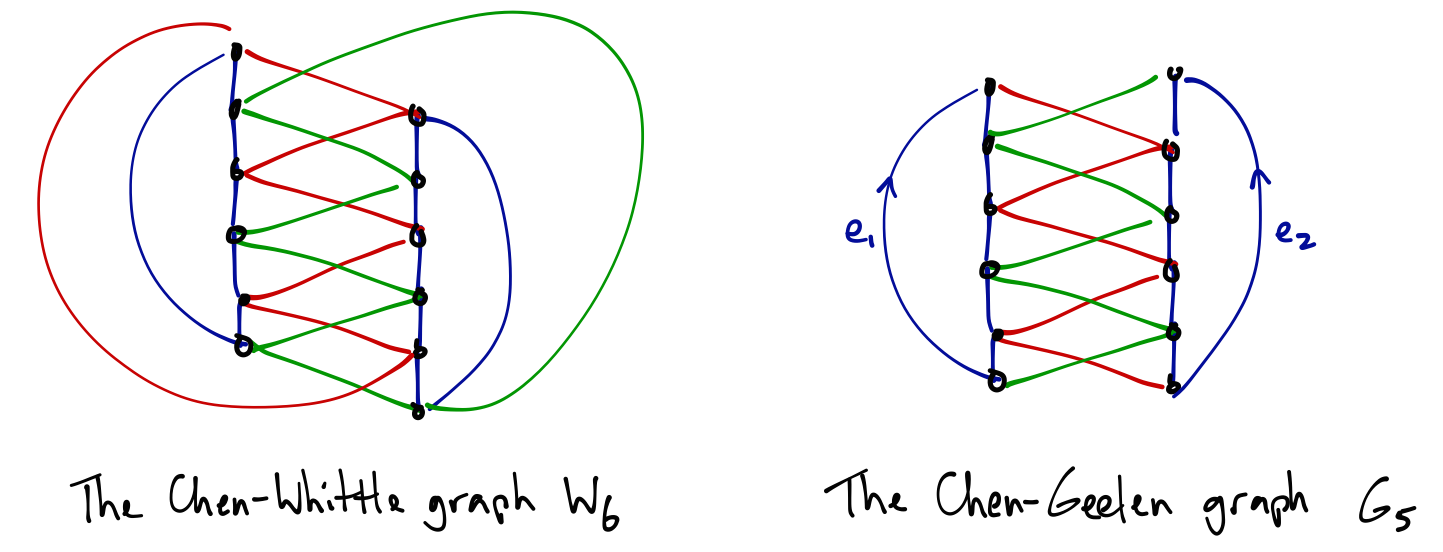Call a green edge and a red edge that cross in this drawing a crossing pair. Observe that $W_k$ has $k$ crossing pairs, and that for every collection $S$ of an even number of crossing pairs, there is a pair of disjoint cycles $C_S^1, C_S^2$ which use precisely these crossing pairs, each of which has length $k$, and which between them traverse all vertices of $W_k$. (See figure below: choosing the 2nd and 4th crossing pair defines the pair of cycles highlighted green and yellow.)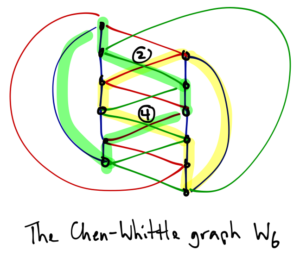The graph $W_k$ has exponentially many collections of crossing pairs: there are $2^{k-1}$ collections consisting of an even number of crossing pairs. Hence $W_k$ has $2^{k-1}$ pairs of disjoint cycles, each pair having the property that together they contain all vertices of $W_k$. Each such pair of cycles is a circuit-hyperplane of the lift matroid $LM(W_k,\emptyset)$, and a free basis of the frame matroid $FM(W_k,\emptyset)$. Let $Z$ be the edge set of a pair of cycles obtained as above from a chosen set of crossing pairs of even cardinality. Let $M_L^Z$ be the matroid obtained from $LM(W_k,\emptyset)$ by relaxing the circuit-hyperplane $Z$. Let $M_F^Z$ be the matroid obtained from $FM(W_k,\emptyset)$ by tightening the free basis $Z$. To distinguish $LM(W_k,\emptyset)$ from $M_L^Z$, and to distinguish $FM(W_k,\emptyset)$ from $M_F^Z$, requires checking the rank of $2^{k-1}$ subsets. This, of course, will be greater than any polynomial in $|E(W_k)|$ for sufficiently large $k$. Since $W_k$ remains a framework for both $M_L^Z$ and $M_F^Z$, both these matroids are quasi-graphic. The proofs are completed by showing that $M_L^Z$ is not a lift matroid, and that $M_F^Z$ is not frame (which takes just another couple of pages in Rong and Geoff’s paper).

The Chen-Whittle graph’s usefulness does not stop here.

# Excluded minors

To disprove Conjectures 1 and 2, Rong and Jim exhibit an infinite family of quasi-graphic matroids, each of which is minor-minimally not frame, and another infinite family of quasi-graphic matroids, each of which is minor-minimally not lifted-graphic. As in Rong and Geoff’s proofs of Theorems 2 and 3, these two families share a common framework. In fact, they again use the Chen-Whittle graphs!

Here is Rong and Jim’s construction. For each odd positive integer $k \geq 5$, let $G_k$ be the graph obtained from the Chen-Whittle graph $W_{k+1}$ defined above by deleting exactly one crossing pair (at right in figure above is shown the Chen-Geelen graph $G_5$). It is convenient to describe the collection of balanced cycles of $G_k$ by group-labelling (group-labelled graphs are described in Irene’s first post on biased graphs, Section 1, 4th bullet point).

For each odd positive integer $k \geq 5$, we obtain a quasi-graphic excluded minor for the class of frame matroids, with framework $G_k$, as follows. Label $G_k$ using the multiplicative group of $\mathbb R$. Referring to the drawing of $G_5$ above: orient each of $e_1$ and $e_2$ “up” from the bottom vertex to the top vertex of the vertical path making up its “side” of the crossing ladder, and assign to both $e_1$ and $e_2$ the label 2. Orient all remaining edges arbitrarily, and assign each label 1. Let $\mathcal B_k$ be the collection of balanced cycles defined by this labelling (that is, ${\mathcal B}_k$ consists of those cycles $C$ for which the product of edge labels, taken while traversing $C$ in a cyclic order, is 1, where we take the multiplicative inverse of each label whose edge is traversed against its orientation). Let $P$ be the paths forming the two vertical sides of the ladder, so $P \cup \{e_1, e_2\}$ is the pair of blue disjoint cycles in the figure, and let $Q$ be the union of the red and green paths. Then $P \cup \{e_1, e_2\}$ and $Q \cup \{e_1, e_2\}$ are free bases of $FM(G_k, \mathcal B_k)$. Let $M_k^F$ be the matroid obtained from $FM(G_k, \mathcal B_k)$ by tightening $P \cup \{e_1, e_2\}$ and $Q \cup \{e_1, e_2\}$. Then $M_k^F/\{e_1, e_2\}$, while quasi-graphic (with framework $G_k/\{e_1,e_2\}$), is an excluded minor for the class of frame matroids.

An excluded minor for the class of lift matroids is obtained in a similar manner. Again orient $e_1$ and $e_2$ up from the bottom to the top vertex of the vertical paths of the ladder. This time, label $G_k$ using elements of the additive group of integers, labelling $e_1$ with $1$, $e_2$ by $-1$, and all remaining edges with $0$. Let $\mathcal B_k$ be the collection of balanced cycles defined by this labelling (that is, $C \in \mathcal B_k$ if and only if when traversing $C$ in a chosen cyclic direction, taking the sum of the labels on its edges, subtracting the label on each edge traversed against its orientation, yields $0$). Let $P$ and $Q$ be as before. Then $P \cup \{e_1,e_2\}$ and $Q \cup \{e_1, e_2\}$ are circuit-hyperplanes of $LM(G_k, \mathcal B_k)$. Let $M_k^L$ be the matroid obtained from $LM(G_k, \mathcal B_k)$ by relaxing $P \cup \{e_1, e_2\}$ and $Q \cup \{e_1, e_2\}$. Then $M_k^L / \{e_1, e_2\}$, while quasi-graphic, is an excluded minor for the class of lift matroids.

Rong and Jim make the following conjecture.

Conjecture 3 [CG17]. The class of quasi-graphic matroids has only a finite number of excluded minors.

Dillon Mayhew and I have recently proved the following three theorems.

Theorem 4 [FM17]. The class of frame matroids has only a finite number of excluded minors of any fixed rank.

Theorem 5 [FM17]. The class of lift matroids has only a finite number of excluded minors of any fixed rank.

Theorem 6 [FM17]. The class of quasi-graphic matroids has only a finite number of excluded minors of any fixed rank.

Rong and Jim’s counterexamples to Conjecture 1 and 2 are both infinite families of excluded minors of ever increasing, arbitrarily large rank (if $G$ is a connected framework for a non-graphic quasi-graphic matroid $M$, then the rank of $M$ is $|V(G)|$). Theorems 4 and 5 say that any such collections of excluded minors for these classes must have this property. Theorem 6 provides evidence toward Conjecture 3 — though no more evidence than Theorems 4 and 5, respectively, provide toward Conjectures 1 and 2! What is perhaps interesting about Theorems 4, 5, and 6 is that — in contrast to what we’ve just seen in the results of Rong, Geoff, and Jim above — the same strategy works for all three classes. Dillon and I set out to prove Theorem 4, and having done so, realised that essentially the same proof also gives us Theorems 5 and 6. Perhaps we can take a look at this strategy in a subsequent post.

# References

[CG17] Rong Chen and Jim Geelen. Infinitly many excluded minors for frame matroids and for lifted-graphic matroids. arXiv:1703.04857

[CW16] Rong Chen and Geoff Whittle. On recognising frame and lifted-graphic matroids. arXiv:1601.01791

[FM17] Daryl Funk and Dillon Mayhew. On excluded minors for classes of graphical matroids. Forthcoming.

[GGW17] Jim Geelen, Bert Gerards, and Geoff Whittle. Quasi-graphic matroids. arXiv:1512.03005

[S81] Paul Seymour. Recognizing graphic matroids. Combinatorica (1981). MR602418